https://doi.org/10.5194/wes-8-421-2023
https://doi.org/10.5194/wes-8-421-2023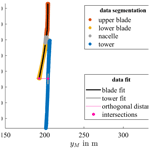# Assessing the rotor blade deformation and tower–blade tip clearance of a 3.4 MW wind turbine with terrestrial laser scanning

Paula Helming, Alex Intemann, Klaus-Peter Webersinke, Axel von Freyberg, Michael Sorg, and Andreas Fischer
Abstract

Share
Dates
1 Introduction

The measurement methods commonly used for this purpose such as strain gauges (Crabtree et al., 2014) or accelerometers (Loh et al., 2017; Oliveira et al., 2018; Ou et al., 2017; Tcherniak and Mølgaard, 2017; Crabtree et al., 2014) often need a wind turbine modification, which leads to high efforts and costs since they have to be installed during a standstill. Also, these sensors measure the deformation only at a few discrete locations, and changing these points of installation means a turbine modification once more.

For this reason, there have been several attempts to contactlessly measure the rotor blade deformation. One of these methods is the use of laser Doppler vibrometry, where the frequency and phase change of a laser beam pointed at the rotor blade is measured to determine the rotor blade oscillations (Ozbek et al., 2009; Baqersad et al., 2017; Dilek et al., 2019). However, the installation of retroflective markers at discrete measuring spots is needed. Another method that could be used without the need of external markers is photogrammetry. Here, the three-dimensional coordinates of the rotor blades are determined from multiple two-dimensional images taken from different positions and orientations by either using point tracking on wind turbine models (Lundstrom et al., 2012), point tracking on actual operating wind turbines (Lundstrom et al., 2012; Ozbek et al., 2010; Ozbek and Rixen, 2013; Ozbek et al., 2013; Winstroth et al., 2014; Lehnhoff et al., 2020; Ozbek and Rixen, 2013; Ozbek et al., 2013) or digital image correlation (DIC) (Winstroth et al., 2014; Lehnhoff et al., 2020). However, several different measurement locations or access points are needed to measure the rotor blade geometry, as well markers on the rotor blade.

Therefore, the aim of the present article is a TLS-based correlation analysis between the applied wind loads on the wind turbine and the rotor blade deformation as well as the tower–blade tip clearance during operation, using neither any prior knowledge of the geometry of the wind turbine nor access to the SCADA data. The correlation analysis is realized by a statistical analysis of the TLS data, which is explained together with the TLS measurement arrangement and principle in Sect. 2. The experimental setup is described in Sect. 3, and the achievable measurement uncertainty is estimated in Sect. 4 by a Monte Carlo simulation. The measurement system is then applied to a 3.4 MW wind turbine, where the deformation of the rotor blade is analyzed for different loads and the measured tower–blade tip clearance is compared to a reference measurement system in Sect. 5; Sect. 6 closes with a summary.

2 Measurement principle and methodology

The TLS used for the present analysis is a scanner in line-scanning mode. The measurement principle and the measuring approach are presented in the following subsections.

## 2.1 Measurement principle

A terrestrial laser scanner (TLS) measures a distance d with the time-of-flight (ToF) principle: a pulsed laser beam is emitted, and the time t it takes for the light to reach the target and return to the laser scanner is measured. With the speed of light c, the distance d is then obtained by calculating

$\begin{array}{}\text{(1)}& d=\frac{\mathrm{c}\cdot t}{\mathrm{2}}.\end{array}$

This distance measurement is repeated while the direction of the emitted laser beam direction is varied, i.e., scanned.

Terrestrial laser scanners in line-scanning mode have a rotating mirror that scans the laser beam over a fixed scan angle θ. Each scan Si, where i is the scan number, is related to a time τi and consists of N measured distances di, which are acquired in incremental steps with a constant angular step width $\mathrm{\Delta }\mathit{\theta }=\frac{\mathit{\theta }}{N}:$

$\begin{array}{}\text{(2)}& {S}_{i}=\left(\begin{array}{c}{d}_{i}\left(\mathrm{\Delta }\mathit{\theta }\right)\\ \mathrm{⋮}\\ {d}_{i}\left(N\phantom{\rule{0.125em}{0ex}}\mathrm{\Delta }\mathit{\theta }\right)\end{array}\right).\end{array}$

As a result, the surface points are first obtained in polar coordinates and, thus, are transformed to two-dimensional Cartesian coordinates (depth axis and scan axis).

## 2.2 Methodology

The laser scanner in line-scanning mode is aligned vertically, scanning the tower and blades of the wind turbine as they pass through the scan line as displayed in Fig. 1.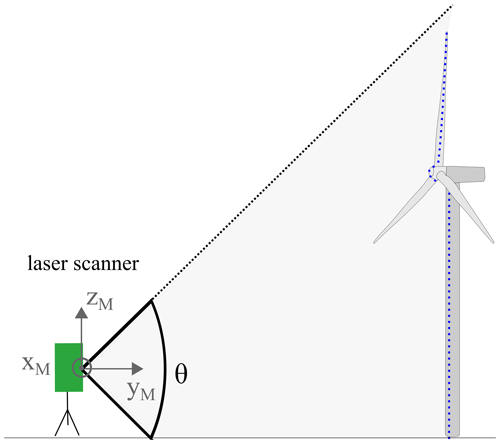Figure 1Sketch of the measurement arrangement: the laser scanner (in green) with the scan angle θ is aligned vertically to the wind turbine. The points that are reflected by the wind turbine (blue dots) are measured in the yM, zM coordinate system.

### 2.2.1 Data segmentation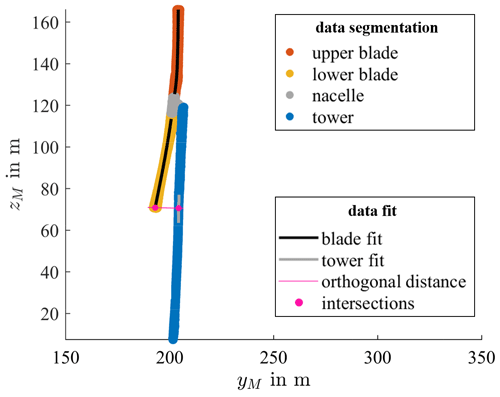Figure 2Results of data segmentation: the assignment of the valid measuring data is color coded according to the legend. Furthermore, the fitting results of the tower and the rotor blade data points are presented, together with the intersections used to calculate the orthogonal distance between the lower-blade tip and the tower.

3 Experimental setup

## 3.1 Measurement object

Measurements are carried out on a 3.4 MW wind turbine of the type REPower 3.4M located in Bremen, Germany. Some of the wind turbine characteristics are summarized in Table 1.

## 3.2 Measurement system and setup

### 3.2.1 Setup

A photo of the experimental setup of the laser scanner aligned vertically to the wind turbine and connected to a measurement laptop is shown in Fig. 3a. The laser is placed upwind from the wind turbine facing it from the front with a distance of 190 m in the first and 200 m in the second experiment. This setup is used for measuring both the rotor blade deformation as well as the tower–blade tip clearance. For validation, the tower–blade tip clearance is simultaneously measured with a camera system as a reference using the method and setup described in He et al. (2013). In contrast to the laser scanner, the video camera is placed at a 90 angle, looking at the wind turbine from the side (see Fig. 3b). The tower–blade tip clearance is then determined by measuring the pixels between the blade and the tower whenever a blade reaches the lowest point; this distance in pixels is then transformed to meters using the known length of the nacelle as a calibration factor. The laser scanner and camera data are then synchronized manually using the displayed time on both devices. Note that no video measurement for the rotor blade deformation experiment was performed.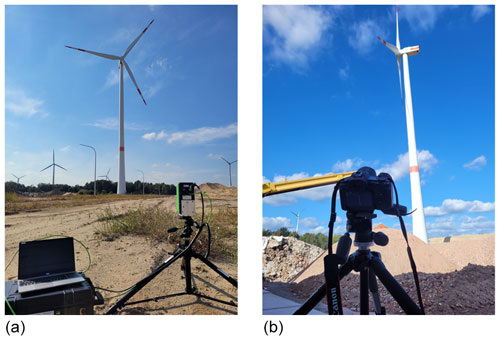Figure 3(a) Experimental setup for field measurements with a laser scanner on a tripod, a measurement laptop and the wind turbine in the background. (b) Experimental setup for field measurements with a camera on a tripod and the wind turbine from the side in the background used as a reference for the tower–blade tip clearance.

### 3.2.2 Measurement system

The laser scanner used in this study is the LASE 2000D-228PLUS using a laser in the infrared range. It produces 1000 measurement points over a scan angle of $\mathit{\theta }=\mathrm{90}{}^{\circ }$, which leads to an angular step width of $\mathrm{\Delta }\mathit{\theta }=\mathrm{0.09}{}^{\circ }$. The scan frequency is 30 Hz. The relevant characteristics of the laser scanner are summarized in Table 2.

The optical camera used for the reference measurements of the tower–blade tip clearance is a Canon EOS R5 system camera; the relevant camera characteristics are summarized in Table 3.

### 3.2.3 Data processing

The data of each scan are saved as a text file and imported into MATLAB, where the data processing is carried out. For the calculation of the rotor speed, all instances of scan Si with measurement points on the blades are considered, even the scans with incomplete blade scan vectors. For each Δθ, where every blade is expected to pass through the scan line, the first point of contact for each blade with the scan line is determined and the time ti of the respective scan Si is recorded. Afterward, the rotor speed is calculated from the time difference Δt between consecutive blades passing through the scan line for each Δθ. For each 10 s interval the calculated rotor speed is averaged, yielding rotor blade speed data with a time resolution of 10 s.

The polynomial fits for both the rotor blade deformation analysis and the tower–blade tip clearance are done as least-squares fittings using the MATLAB plot-fitting toolbox. To calculate the tower–blade tip clearance, the lowest zM coordinate which is present in all blades is used as the point of interest on the tower, and a region ±3 m in zM is used to fit a line through the tower. A further line, which is orthogonal to tower fit curve and passes through the point of interest, is constructed intersecting the blade fit. The length between the point of interest and the intersection point is defined as the tower–blade tip clearance. Note that the local thickness should be subtracted from the tower–blade tip clearance, which is not applied here since the thickness is unknown and the focus of this study is the analysis of the clearance fluctuations. The data for the tower–blade tip clearance are smoothened using a moving median with a window size of 3 s.

## 3.3 Measurement conditions

The measurement conditions for the two measurement campaigns, where each was performed regarding one of the two measurement tasks (rotor blade deformation, tower–blade tip clearance), are summarized in Table 4. Note that the wind speed displayed here is only a rough estimate of the average wind speed with an unknown uncertainty since we do not have access to an external wind lidar measurement system or detailed SCADA data. Both measurements took place for around 30 min. For the last 18 min of the tower–blade tip clearance measurement, the clearance was also measured with the reference camera measurement system. The measurements were carried out during partial-load operation mode of the wind turbine to avoid influences of pitching of the rotor blades in the data. No yaw movement occurred during the measurement period.

4 Uncertainty analysis

## 4.1 Uncertainty in the laser scanner

To determine the uncertainty for one measurement point of the laser scanner, a whiteboard with reflective characteristics similar to the rotor blade is placed at distance s from 50 to 300 m with a step size of 50 m (see Fig. 4). The board is also scanned at 310 m, which is the largest specified measuring distance with the used laser scanner. Additionally, measurements with different instances of tilt angle γ of the board are performed, i.e., for $\mathit{\gamma }=\mathrm{0}{}^{\circ }$, $\mathit{\gamma }=\mathrm{15}{}^{\circ }$ and $\mathit{\gamma }=\mathrm{30}{}^{\circ }$. For each constellation, i>10 000 scans are conducted, and the standard deviation of one point dθ) is calculated. Note that the systematic error in the laser scanner is not considered, since not the absolute distance measurements but only the distance changes or a relative distance with respect to the measured distance at a different scan position is of interest. Thus, the standard deviation yields the random error in one point of the laser scanner, which is studied here in dependency of the working distance.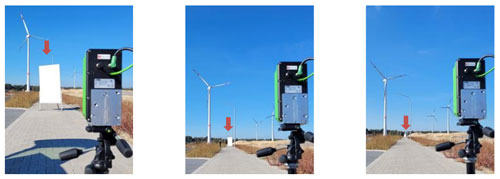Figure 4Whiteboard (marked with red arrow) placed at different working distances and with different angles with respect to the laser scanner. The number of scans for each position and orientation amounts to >10 000.

Figure 5 shows the calculated standard deviation over the working distance s for the tilt angle $\mathit{\gamma }=\mathrm{0}{}^{\circ }$ in blue, $\mathit{\gamma }=\mathrm{15}{}^{\circ }$ in red and $\mathit{\gamma }=\mathrm{30}{}^{\circ }$ in yellow, respectively. A linear polynomial function is fitted through each dataset and is represented as a dotted line. The standard deviation increases linearly with the distances as described in Freyberg et al. (2021) and increases with an increased angle γ.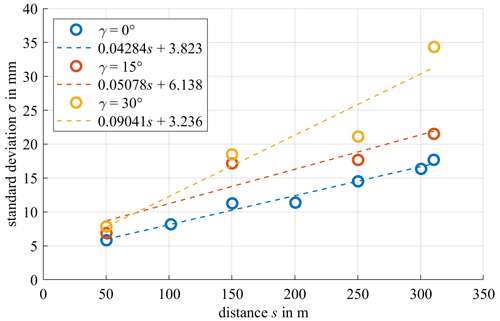Figure 5Standard deviation of the measured distance to a whiteboard over the working distance s for different board tilt angles γ (circles). A line is fitted through the points (dotted lines).

## 4.2 Uncertainty in rotor blade deformation fit

In order to determine the uncertainty in a rotor blade fit concerning the rotor blade deformation analysis, the determined behavior of the standard deviation of a single measurement point from Fig. 5 is now used to perform a Monte Carlo simulation according to Joint Committee for Guides in Metrology (2008). Due to the twists in the rotor blade, it is hardly possible to predict the exact angle between the laser beam and the rotor blade surface. Therefore, three different Monte Carlo simulations are carried out, each assuming that the rotor blade is hit by the laser beam at a constant angle, i.e., $\mathit{\gamma }=\mathrm{0}{}^{\circ }$, $\mathit{\gamma }=\mathrm{15}{}^{\circ }$ or $\mathit{\gamma }=\mathrm{30}{}^{\circ }$. As the basis for one run of the Monte Carlo simulation, 92 measuring points are simulated to represent the upper blade and 83 measuring points are simulated to represent the lower blade, which is equivalent to the number of points in the actual blade measurement that is contained in one scan. Normally distributed noise is added to each data point according to its nominal distance to the laser scanner. To complete one run of the Monte Carlo simulation, this generation of simulated measurement data is repeated 263 times, and an example of the resulting data set is shown in Fig. 6 (blue circles). The number of data is equivalent to the number of blades measured in the measurement period for the category of the higher rotational wind speeds ω>ωmean. Note that the simulation does not take effects from the blades' movement into account. Through all simulated measuring points (92×263 for the upper blade and 83×263 for the lower blade), a fourth-degree polynomial function is fitted through the points, separately for the upper and the lower blade (black lines in Fig. 6), as described in the method in Sect. 2.2.2.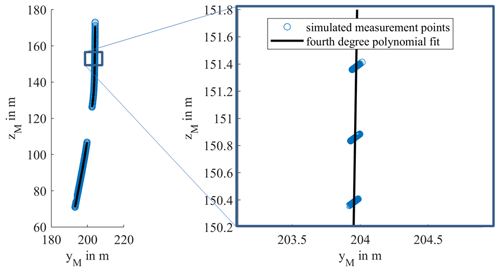Figure 6Simulated points on blades for 263 scans with noise added to each simulated measuring point (blue circles) for the case $\mathit{\gamma }=\mathrm{0}{}^{\circ }$. A fourth-degree polynomial is fitted through the simulated points (black line).

One run of this simulation is repeated 10 000 times, and the standard deviation of the fit in the yM direction as a function of zM is calculated and shown in Fig. 7. The standard deviation increases with an increasing tilt angle γ. The standard deviation is fairly constant in the middle of the blades but increases rapidly at the end points of the blades. Due to the divergence of high-order polynomial functions outside of the interval considered for interpolation over a set of equispaced points (see Boyd and Xu, 2009), the residue of a least-squares approximation in turn increases at the edges of the fit interval, leading to a rapidly increasing standard deviation for points approaching the edges of the blades as seen in Fig. 7. If the start and end points of the blades are ignored, the uncertainty in the fit resulting from the uncertainty in the laser scanner is below 0.3 mm, even in the scenario where the laser beam hits the rotor blade at an angle of $\mathit{\gamma }=\mathrm{30}{}^{\circ }$; even with the endpoints included it reaches a maximum of less than 0.7 mm. Using the laser scanner, it is therefore possible to differentiate between two deformed states in the millimeter range.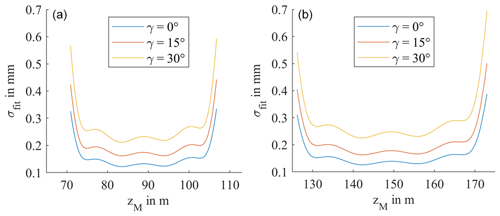Figure 7Standard deviation of the fourth-degree polynomial fit along the zM axis for the (a) lower blade and (b) upper blade through 263 simulated rotor blade scans repeated 10 000 times.

## 4.3 Uncertainty tower–blade tip clearance

To determine the uncertainty in the rotor blade fit for the tower–blade tip clearance, another three Monte Carlo simulations are carried out with different instances of angle γ. Similar to Sect. 4.2, rotor blades are simulated, but only the measurement points corresponding to the lower blade are simulated and added with noise (see Fig. 8). Additionally, the measurement points for one tower scan are also simulated and added with normally distributed noise. As described in Sect. 2.2.3, a fourth-degree polynomial is fitted through the simulated blade measurement, a line is fitted through the tower measurement and the line intersecting both fits is calculated to finally obtain a measure of the tower–blade tip clearance. This is repeated 10 000 times, and the standard deviation of this calculated tower–blade tip clearance is computed and shown in Fig. 9. The uncertainty is a magnitude higher compared to the uncertainty in the blade deformation fit and increases with an increasing angle γ. This can be explained by the fact that only the simulated measurement points of one blade and one tower scan are used for this calculation, which increases the uncertainty. Additionally, the tower–blade tip clearance uses the tip of the rotor blade fit, which has the highest uncertainty as shown in Sect. 4.2. Nevertheless, the tower–blade tip clearance can be calculated with an uncertainty of around 1 cm, even when the laser scanner hits the surface at an angle of $\mathit{\gamma }=\mathrm{30}{}^{\circ }$.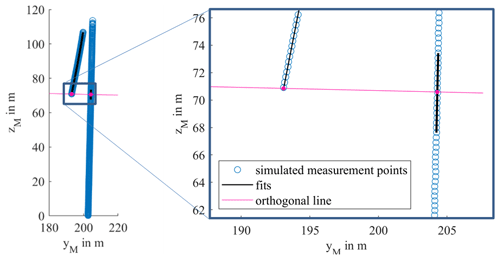Figure 8Simulated measuring points on the blade and tower for one scan with noise added to the blade and tower points (blue circles) for the case $\mathit{\gamma }=\mathrm{0}{}^{\circ }$ with fits through simulated points in black. The orthogonal distance with the tower and blade is shown in pink.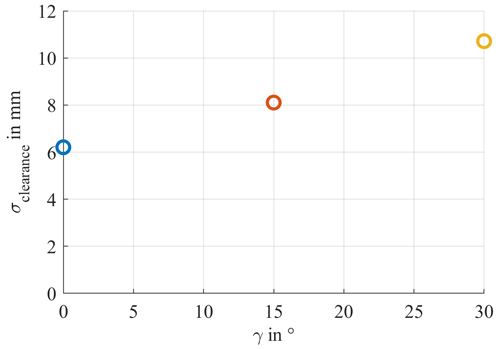Figure 9Standard deviation of the simulated tower–blade tip clearance from 10 000 repetitions for different instances of angle γ.

5 Measurement results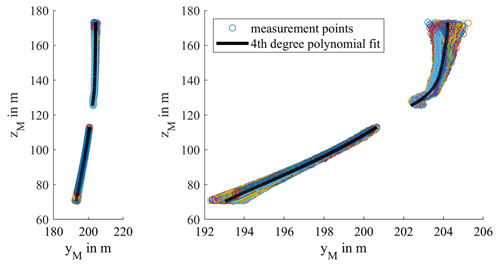Figure 10Measurement points on the blades as circles and fourth-degree polynomial fits for blade 1 for all rotor speeds.

In order to analyze the rotor blade deformation for different wind loads, the scans are sorted according to the rotor speed at the time, and a fourth-degree polynomial is fitted separately through all measured blade points for each category, as described in Sects. 2.2.2. and 3.2.3. The measured rotor speed ω is shown over the measurement time in Fig. 12 with the mean rotor speed ωmean shown as a dotted line. To show the difference between higher wind load and lower wind load, each scan is sorted as either (a) higher (shaded in orange) or (b) lower or equal (shaded in blue) than the mean rotational speed ωmean=8.6 rpm.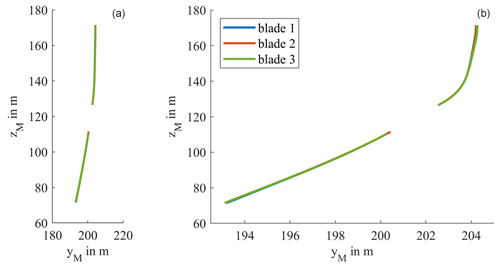Figure 11Blade deformation for different blades: fourth-degree polynomial fitted through all measurement points of the separate blades with (a) equal spacing between the yM and zM axis and (b) distorted view.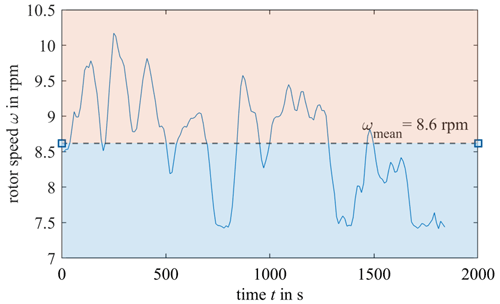Figure 12Measured rotor speed ω over the measurement time with ωmean=8.6 rpm. Each scanned rotor blade is categorized into higher wind loads (ω>ωmean) shaded in orange or lower wind loads (ωωmean) shaded in blue.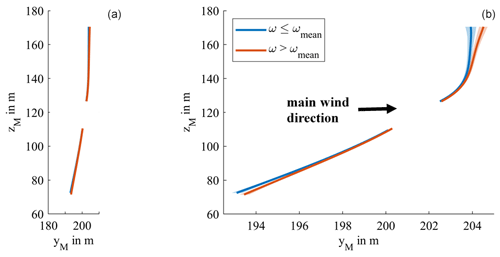Figure 13Blade deformation for different wind loads for blade 1: fourth-degree polynomial fitted through all measurement points on the blades for higher wind load (ω>ωmean) in orange and lower wind loads (ωωmean) in blue, respectively, with (a) equal spacing between the yM and zM axis and (b) distorted view.

The tower–blade tip clearance is determined as described in Sect. 2.2.3 and, as a validation, a video measurement system also measuring tower–blade tip clearance from the side is used for the second half of the measurement period ( 1100 s). In Fig. 13, both the tower–blade tip clearances calculated from the laser scanner system and the video system are shown over the measurement period in seconds. Note that the graph consists of discrete points with a time interval of 1–2 s. However, they are shown here as continuous in order to improve visualization. The laser-based tower–blade tip clearance measurement shows good agreement with the video-based reference measurement, deviating only 0.06 m or 0.06 % on average with a standard deviation of 0.175 m or 1.7 % from the mean tower–blade tip clearance. The laser scanner is therefore an efficient measurement system to evaluate the tower–blade tip clearance. The difference between the minimum and the maximum measured clearance is around 1.5 m, which is significantly higher than the simulated uncertainty of 0.01 m for the laser scanner. The deviation between the laser scanner and the video system can be at least partly explained by the uncertainty in the video measurement system.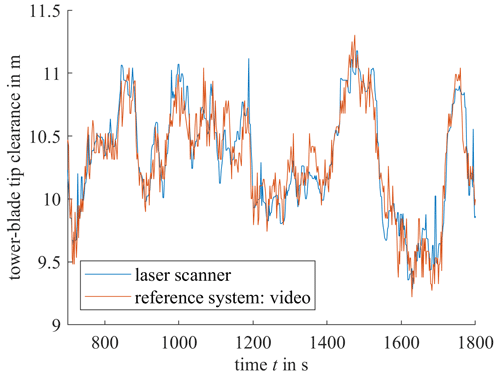Figure 14Tower–blade tip clearance over time with a laser scanner and video reference measurement system. Note that the graph consists of discrete points with a time interval of 1–2 s. However, they are shown here as continuous in order to improve visualization.

In addition to the tower–blade tip clearance, the rotor speed ω is calculated at the same time. Figure 15 shows the measured tower–blade tip clearance from the laser scanner for the different blades (blade 1 in blue, blade 2 in orange, blade 3 in green) over the associated calculated rotor speed ω for the whole measurement period ( 1800 s). Since the rotor speed can be considered a measure of the wind speed (partial-load operation), the diagram shows the relation between tower–blade tip clearance and wind load.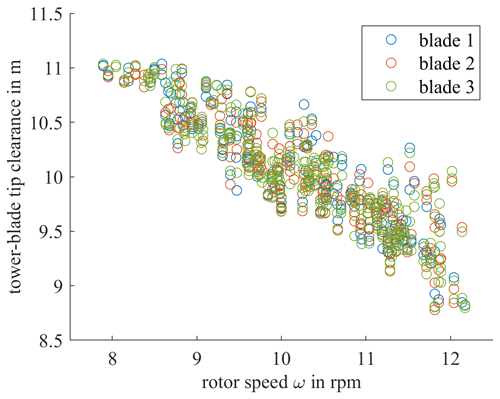Figure 15Tower–blade tip clearance over rotor speed ω with a laser scanner system for different blades (blade 1 in blue, blade 2 in orange and blade 3 in green).

While no difference is visible in the behavior between the three blades, a relatively high deviation occurs, which could be caused by 10 s averaging while calculating the rotor speed. However, there is a clear correlation between the measured tower–blade tip clearance and the calculated rotor speed: the clearance decreases with rising rotor speed as the rising wind force pushes the blade towards the tower and thus decreases the distance between the two.

6 Conclusions

The proposed laser-scanner-based measurement system is capable of comparing the deformed rotor blades for different wind load scenarios, as well as measuring the tower–blade tip clearance as a function of the rotor speed. This was achieved by placing the laser scanner in vertical alignment at a measuring distance of over 150 m from the wind turbine from a single access point. Using the rotor speed as a proxy for the wind load and with the help of a least-squares fitting through the measured rotor blade data points and the measured tower points, it was possible to determine the average deformed rotor blade for different wind loads, as well as the tower–blade tip clearance. The rotor speed was calculated using only the laser scanner data, eliminating the need of an additional measurement device.

The measurement approaches were tested on an operating tall onshore wind turbine in partial-load operation mode. As a result, no behavioral difference between the blades was detected. However, there was a clear correlation between the wind speed and the deformation of the blade as well as the tower–blade tip clearance. To determine the uncertainty in the measurement approach, a Monte Carlo simulation was carried out, where it was shown that a distinction of blade deformations is possible in the millimeter range. The measured differences between the different wind load scenarios were significantly higher than the estimated uncertainty caused from the laser scanner. The measured data from the laser scanner for the tower–blade tip clearance were validated by reference measurements with a video measurement system.

Currently, the analysis between the wind loads and the deformation or tower–blade tip clearance is only possible during partial-load operation mode. By using additional data such as SCADA data or an external wind measurement system, however, the measurement approach can straightforwardly be adopted to also measure in full-load operation mode. Additionally, by using a laser scanner with a smaller scan angle or more scan points, the uncertainty in the measurement could be drastically reduced. The proposed laser scanning method therefore proved to be a capable tool for the structural health monitoring of wind turbine blades.

Code and data availability

The data and code that support the findings of this study are available from the corresponding author, Paula Helming, upon reasonable request.

Author contributions

PH, MS, AF and AvF: conceptualization. PH, AI, AF and KW: methodology. PH and KW: investigation. AI and PH: formal analysis. PH and AI: software. PH: writing (original draft preparation). AvF, MS and AF: writing (review and editing). MS and AF: supervision.

Competing interests

The contact author has declared that none of the authors has any competing interests.

Disclaimer

Publisher's note: Copernicus Publications remains neutral with regard to jurisdictional claims in published maps and institutional affiliations.

Financial support

This research has been supported by the Bundesministerium für Wirtschaft und Klimaschutz (PreciWind (grant no. 03EE3013A)).

The article processing charges for this open-access publication were covered by the University of Bremen.

Review statement

This paper was edited by Weifei Hu and reviewed by S. Artese and one anonymous referee.

References

Artese, S. and Nico, G.: TLS and GB-RAR Measurements of Vibration Frequencies and Oscillation Amplitudes of Tall Structures: An Application to Wind Towers, APPL SCI-BAS, 10, 2237, https://doi.org/10.3390/app10072237, 2020.

Baqersad, J., Poozesh, P., Niezrecki, C., and Avitabile, P.: Photogrammetry and optical methods in structural dynamics – A review, MECH SYST SIGNAL PR, 86, 17–34, https://doi.org/10.1016/j.ymssp.2016.02.011, 2017.

Boyd, J. P. and Xu, F.: Divergence (Runge Phenomenon) for least-squares polynomial approximation on an equispaced grid and Mock–Chebyshev subset interpolation, Appl. Math. Comput., 210, 158–168, https://doi.org/10.1016/j.amc.2008.12.087, 2009.

Crabtree, C. J., Zappalá, D., and Tavner, P. J.: Survey of commercially available condition monitoring systems for wind turbines, Technical Report, Durham University School of Engineering and Computing Sciences and the SUPERGEN Wind Energy Technologies Consortium, Durham. Univ. J., https://dro.dur.ac.uk/12497/ (last acesss: 20 October 2022), 2014.

Dilek, A. U., Oguz, A. D., Satis, F., Gokdel, Y. D., and Ozbek, M.: Condition monitoring of wind turbine blades and tower via an automated laser scanning system, Eng. Struct, 189, 25–34, https://doi.org/10.1016/j.engstruct.2019.03.065, 2019.

Freyberg, A. von, Helming, P., Friedrich, J., Stöbener, D., and Fischer, A.: Non-contact measurement of wind turbine rotor blade pitch angle deviations from 150 m distance, TM – Tech. Mess., 88, 686–695, https://doi.org/10.1515/teme-2021-0085, 2021.

Goering, M. and Luhmann, T.: Development of a fan-shaped distance meter system for measuring moving rotor blades – concept, photogrammetric orientation and first results, ISPRS Annals of the Photogrammetry, Remote Sensing and Spatial Information Sciences, https://doi.org/10.5194/isprs-annals-V-2-2020-711-2020, 2020.

Grosse-Schwiep, M., Piechel, J., and Luhmann, T.: Measurement of Rotor Blade Deformations of Wind Energy Converters with Laser Scanners, J. Phys. Conf. Ser., 524, 12067, https://doi.org/10.1088/1742-6596/524/1/012067, 2014.

He, L., Qiu, H., Fu, X., and Wu, Z.: Camera-based portable system for wind turbine blade tip clearance measurement, in: 2013 IEEE International Conference on Imaging Systems and Techniques (IST), Beijing, China, 22–23 October 2013, https://doi.org/10.1109/IST.2013.6729740, 2013.

Helming, P., von Freyberg, A., Sorg, M., and Fischer, A.: Wind Turbine Tower Deformation Measurement Using Terrestrial Laser Scanning on a 3.4 MW Wind Turbine, Energies, 14, 3255, https://doi.org/10.3390/en14113255, 2021.

Hoghooghi, H., Chokani, N., and Abhari, R. S.: Optical measurements of multi-megawatt wind turbine dynamic response, J. Wind Eng. Ind. Aerod., 206, 104214, https://doi.org/10.1016/j.jweia.2020.104214, 2020.

Joint Committee for Guides in Metrology: JCGM 100:2008 Evaluation of measurement data – Supplement 1 to the GUM – Propagation of distributions using a Monte Carlo method, 17.020 – Metrology and measurement in general, 90 pp., 2008.

Lehnhoff, S., Gómez González, A., and Seume, J. R.: Full-scale deformation measurements of a wind turbine rotor in comparison with aeroelastic simulations, Wind Energ. Sci., 5, 1411–1423, https://doi.org/10.5194/wes-5-1411-2020, 2020.

Loh, C.-H., Loh, K. J., Yang, Y.-S., Hsiung, W.-Y., and Huang, Y.-T.: Vibration-based system identification of wind turbine system, Struct. Control. Hlth., 24, e1876, https://doi.org/10.1002/stc.1876, 2017.

Lundstrom, T., Baqersad, J., Niezrecki, C., and Avitabile, P.: Using High-Speed Stereophotogrammetry Techniques to Extract Shape Information from Wind Turbine/Rotor Operating Data, in: Topics in Modal Analysis II, Volume 6, Springer, New York, NY, 269–275, https://doi.org/10.1007/978-1-4614-2419-2_26, 2012.

Oliveira, G., Magalhães, F., Cunha, Á., and Caetano, E.: Vibration-based damage detection in a wind turbine using 1 year of data, Struct. Control. Hlth., 25, e2238, https://doi.org/10.1002/stc.2238, 2018.

Ou, Y., Chatzi, E. N., Dertimanis, V. K., and Spiridonakos, M. D.: Vibration-based experimental damage detection of a small-scale wind turbine blade, Struct. Health Monit., 16, 79–96, https://doi.org/10.1177/1475921716663876, 2017.

Ozbek, M. and Rixen, D. J.: Operational modal analysis of a 2.5 MW wind turbine using optical measurement techniques and strain gauges, Wind Energy, 16, 367–381, https://doi.org/10.1002/we.1493, 2013.

Ozbek, M., Rixen, D. J., and Verbruggen, T. W.: Remote monitoring of wind turbine dynamics by laser interferometry phase 1, Proceedings of the IMAC-XXVII: a conference & exposition on structural dynamics 2009, 1–16, https://research.tudelft.nl/en/publications/remote-monitoring-of-wind-turbine-dynamics-by-laser-interferometr (last access: 20 October 2022), 2009.

Ozbek, M., Rixen, D. J., Erne, O., and Sanow, G.: Feasibility of monitoring large wind turbines using photogrammetry, Energy, 35, 4802–4811, https://doi.org/10.1016/j.energy.2010.09.008, 2010.

Ozbek, M., Meng, F., and Rixen, D. J.: Challenges in testing and monitoring the in-operation vibration characteristics of wind turbines, Mech. Syst. Signal Pr., 41, 649–666, https://doi.org/10.1016/j.ymssp.2013.07.023, 2013.

Schill, F. and Eichhorn, A. (Eds.): Investigations of low- and high-frequency movements of wind power plants using a profile laser scanner, 3rd Joint International Symposium on Deformation Monitoring (JISDM), Vienna, Austria, 30 March–1 April 2016, Vol. 30, 2016.

Tcherniak, D. and Mølgaard, L. L.: Active vibration-based structural health monitoring system for wind turbine blade: Demonstration on an operating Vestas V27 wind turbine, Struct. Health Monit., 16, 536–550, https://doi.org/10.1177/1475921717722725, 2017.

Winstroth, J., Schoen, L., Ernst, B., and Seume, J. R.: Wind turbine rotor blade monitoring using digital image correlation: a comparison to aeroelastic simulations of a multi-megawatt wind turbine, J. Phys. Conf. Ser., 524, 12064, https://doi.org/10.1088/1742-6596/524/1/012064, 2014.